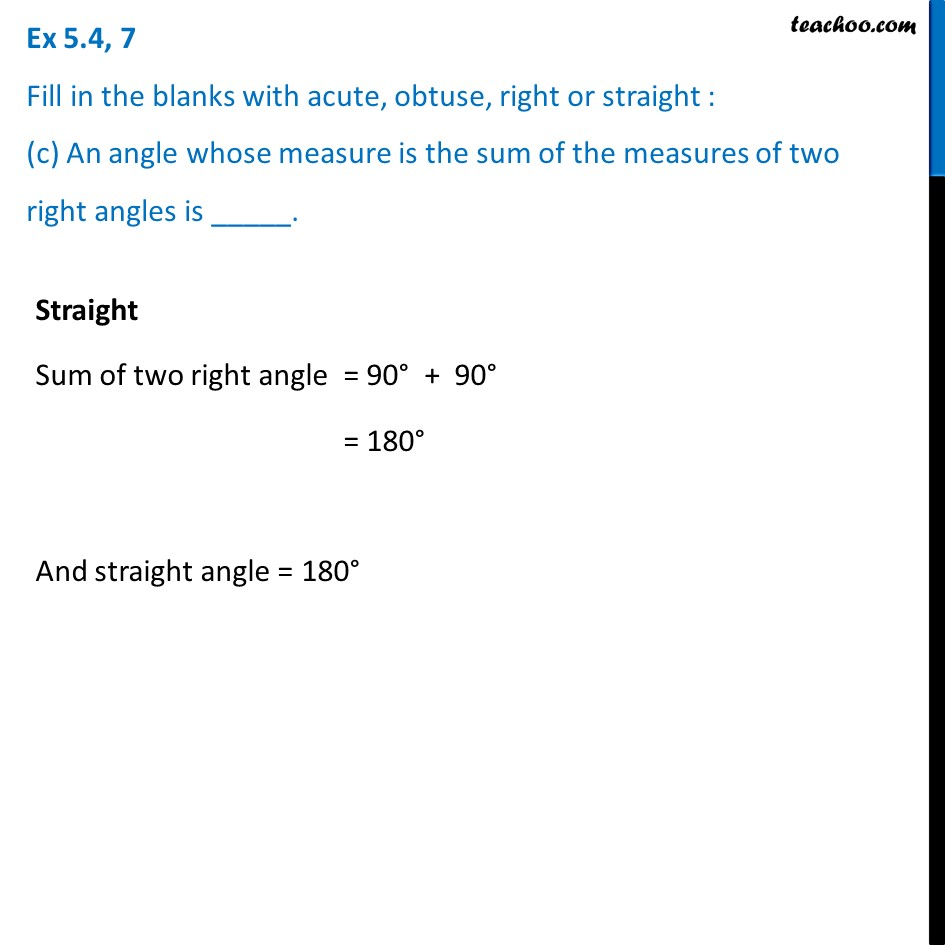1. Chapter 5 Class 6 Understanding Elementary Shapes
2. Serial order wise
3. Ex 5.4

Transcript

Ex 5.4, 7 Fill in the blanks with acute, obtuse, right or straight : (c) An angle whose measure is the sum of the measures of two right angles is _____. Straight Sum of two right angle = 90° + 90° = 180° And straight angle = 180°